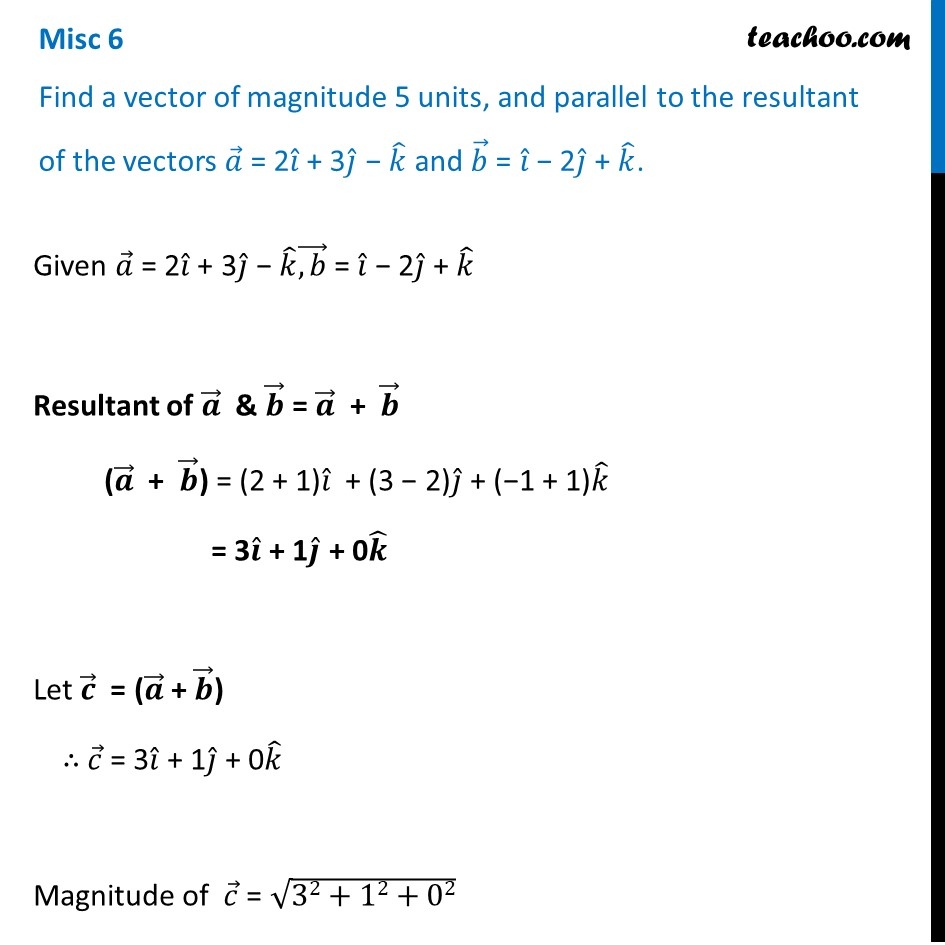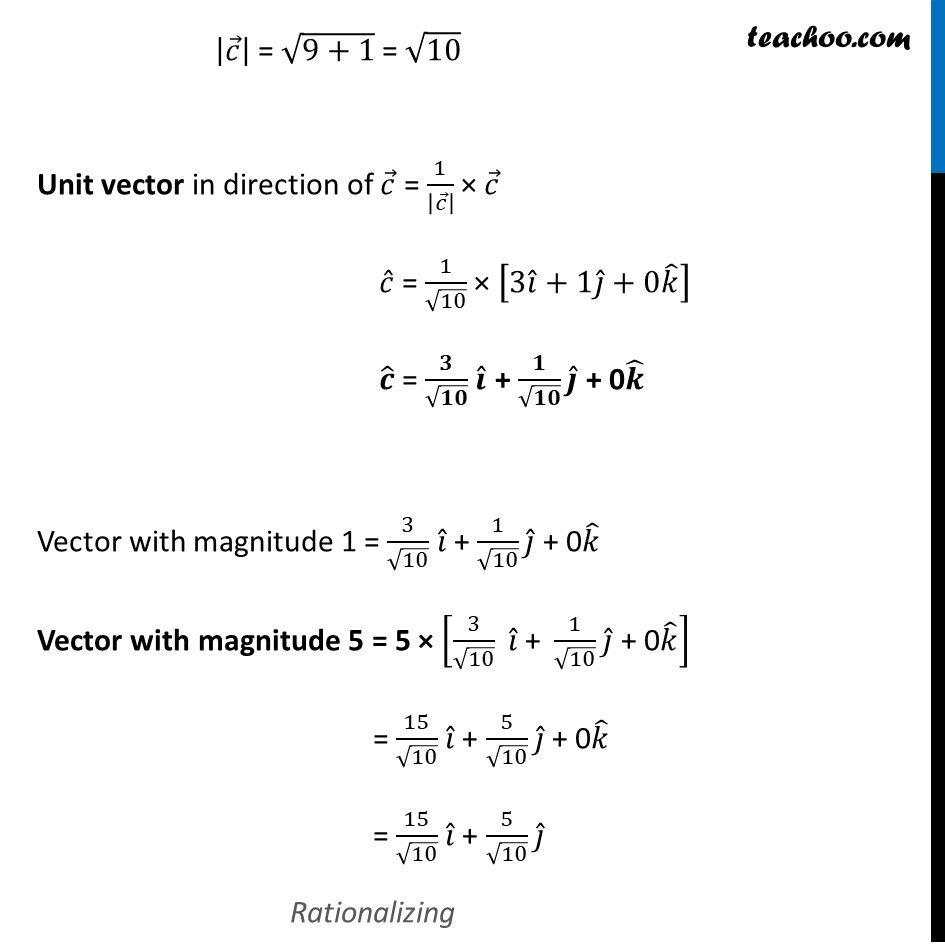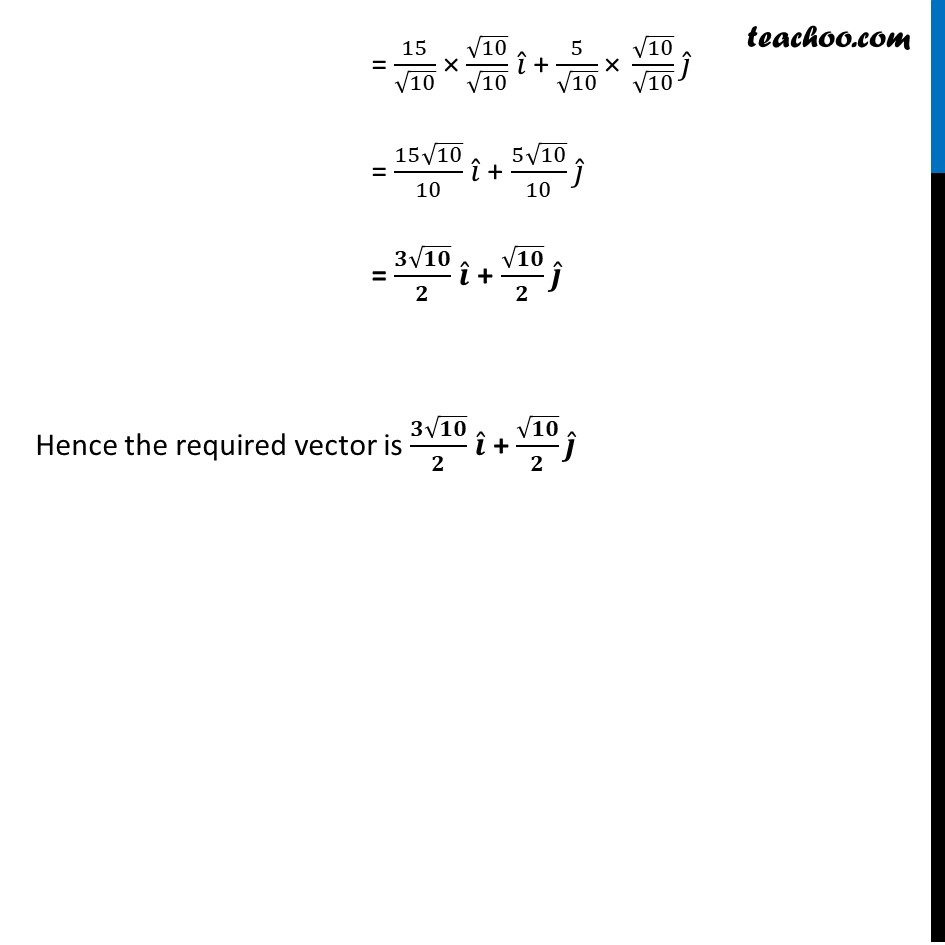Miscellaneous

Chapter 10 Class 12 Vector Algebra
Serial order wiseLearn in your speed, with individual attention - Teachoo Maths 1-on-1 Class

### Transcript

Misc 6 Find a vector of magnitude 5 units, and parallel to the resultant of the vectors 𝑎 ⃗ = 2𝑖 ̂ + 3𝑗 ̂ − 𝑘 ̂ and 𝑏 ⃗ = 𝑖 ̂ − 2𝑗 ̂ + 𝑘 ̂.Given 𝑎 ⃗ = 2𝑖 ̂ + 3𝑗 ̂ − 𝑘 ̂(, 𝑏) ⃗ = 𝑖 ̂ − 2𝑗 ̂ + 𝑘 ̂ Resultant of 𝒂 ⃗ & 𝒃 ⃗ = 𝒂 ⃗ + 𝒃 ⃗ (𝒂 ⃗ + 𝒃 ⃗) = (2 + 1)𝑖 ̂ + (3 − 2)𝑗 ̂ + (−1 + 1)𝑘 ̂ = 3𝒊 ̂ + 1𝒋 ̂ + 0𝒌 ̂ Let 𝒄 ⃗ = (𝒂 ⃗ + 𝒃 ⃗) ∴ 𝑐 ⃗ = 3𝑖 ̂ + 1𝑗 ̂ + 0𝑘 ̂ Magnitude of 𝑐 ⃗ = √(32+12+02) |𝑐 ⃗ | = √(9+1) = √10 Unit vector in direction of 𝑐 ⃗ = 1/|𝑐 ⃗ | × 𝑐 ⃗ 𝑐 ̂ = 1/√10 × [3𝑖 ̂+1𝑗 ̂+0𝑘 ̂ ] 𝒄 ̂ = 𝟑/√𝟏𝟎 𝒊 ̂ + 𝟏/√𝟏𝟎 𝒋 ̂ + 0𝒌 ̂ Vector with magnitude 1 = 3/√10 𝑖 ̂ + 1/√10 𝑗 ̂ + 0𝑘 ̂ Vector with magnitude 5 = 5 × [3/√10 " " 𝑖 ̂" + " 1/√10 𝑗 ̂" + 0" 𝑘 ̂ ] = 15/√10 𝑖 ̂ + 5/√10 𝑗 ̂ + 0𝑘 ̂ = 15/√10 𝑖 ̂ + 5/√10 𝑗 ̂ Rationalizing = 15/√10 × √10/√10 𝑖 ̂ + 5/√10 "× " √10/√10 𝑗 ̂ = (15√10)/10 𝑖 ̂ + (5√10)/10 𝑗 ̂ = (𝟑√𝟏𝟎)/𝟐 𝒊 ̂ + √𝟏𝟎/𝟐 𝒋 ̂ Hence the required vector is (𝟑√𝟏𝟎)/𝟐 𝒊 ̂ + √𝟏𝟎/𝟐 𝒋 ̂